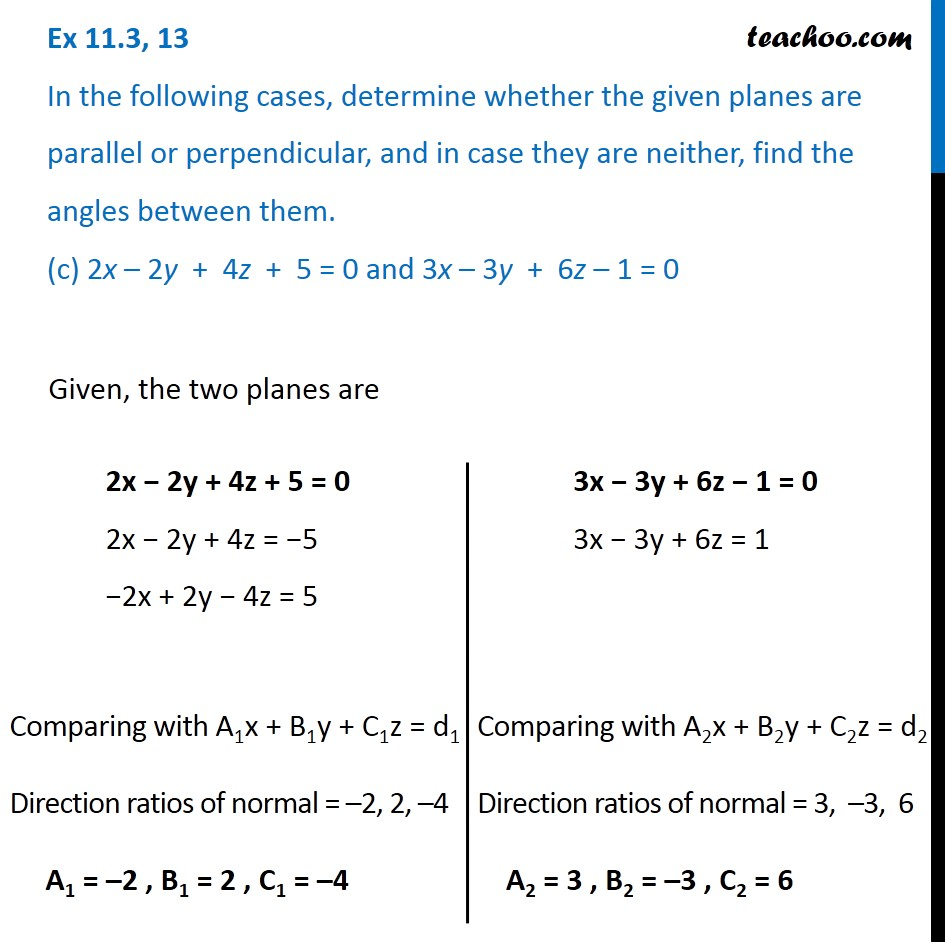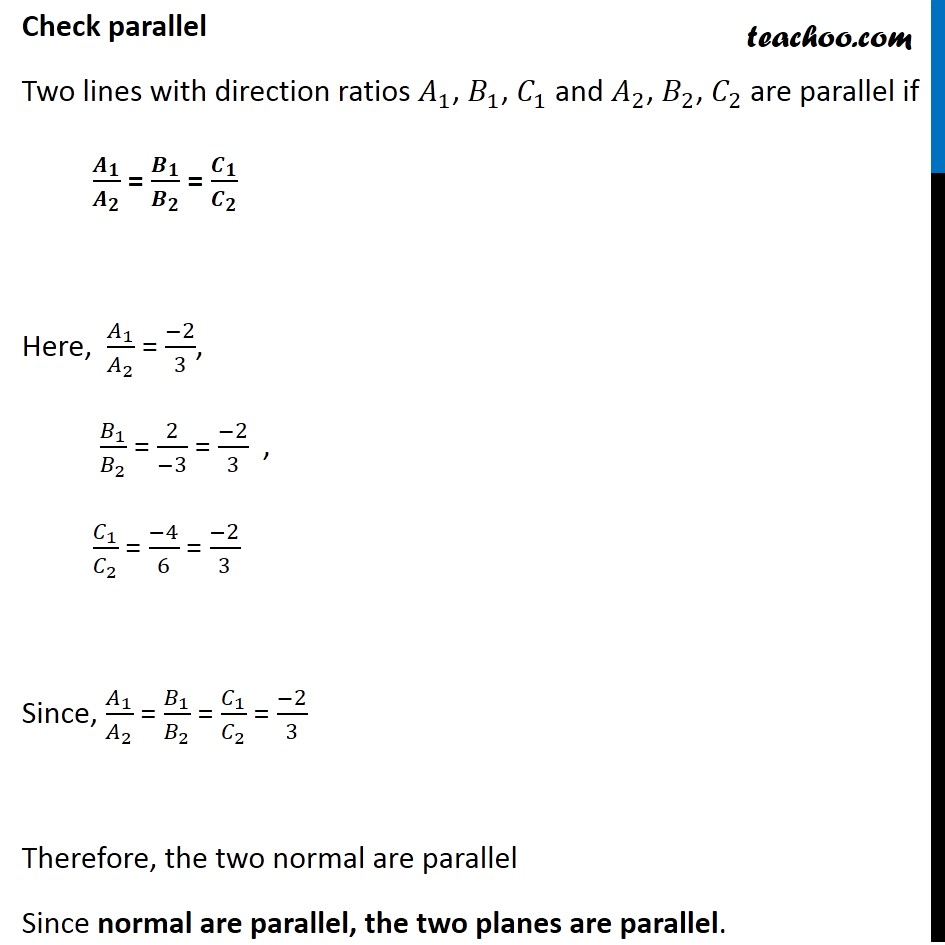Ex 11.3

Chapter 11 Class 12 Three Dimensional Geometry
Serial order wiseGet live Maths 1-on-1 Classs - Class 6 to 12

### Transcript

Ex 11.3, 13 In the following cases, determine whether the given planes are parallel or perpendicular, and in case they are neither, find the angles between them. (c) 2x – 2y + 4z + 5 = 0 and 3x – 3y + 6z – 1 = 0 2x − 2y + 4z + 5 = 0 2x − 2y + 4z = −5 −2x + 2y − 4z = 5 Comparing with A1x + B1y + C1z = d1 Direction ratios of normal = –2, 2, –4 A1 = –2 , B1 = 2 , C1 = –4 3x − 3y + 6z − 1 = 0 3x − 3y + 6z = 1 Comparing with A2x + B2y + C2z = d2 Direction ratios of normal = 3, –3, 6 A2 = 3 , B2 = –3 , C2 = 6 Check parallel Two lines with direction ratios 𝐴_1, 𝐵_1, 𝐶_1 and 𝐴_2, 𝐵_2, 𝐶_2 are parallel if 𝑨_𝟏/𝑨_𝟐 = 𝑩_𝟏/𝑩_𝟐 = 𝑪_𝟏/𝑪_𝟐 Here, 𝐴_1/𝐴_2 = (−2)/3, 𝐵_1/𝐵_2 = 2/(−3) = (−2)/3 , 𝐶_1/𝐶_2 = (−4)/6 = (−2)/3 Since, 𝐴_1/𝐴_2 = 𝐵_1/𝐵_2 = 𝐶_1/𝐶_2 = (−2)/3 Therefore, the two normal are parallel Since normal are parallel, the two planes are parallel.